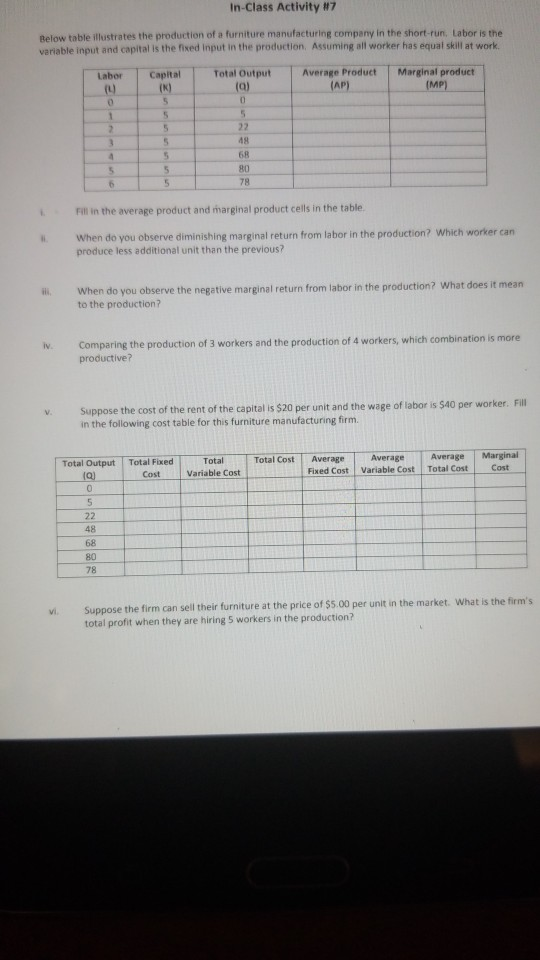Homework Help Question & Answers

In-class Activity #7 Below table illustrates the production of a furniture manufacturing company ...

How to solve for the tableIn-class Activity #7 Below table illustrates the production of a furniture manufacturing company in the short-run, Labor is the variable input and capital is the fixed Input in the production. Assuming all worker has equal skill at work. Average Product-T Marginal product Total output Labor Capital 48 68 80 78 Fill in the average product and marginal product cells in the table. When do you observe diminishing marginal return from labor in the production? Which worker can produce less additional unit than the previous? When do you observe the negative marginal return from labor in the production? What does it mean ii. to the production? iv. Comparing the production of 3 workers and the production of 4 workers, which combination is more productive? . Suppose the cost of the rent of the capital is \$20 per unit and the wage of labor is \$40 per worker. Fill in the following cost table for this furniture manufacturing firm. AverageAverageMarginal Total Total Cost Average Total Output Total Fixed Cost Fixed Cost Variable Cost Total Cost C Cost 48 68 80 78 the firm can sell their furniture at the price of \$5.00 per unit in the market. What is the firm's total profit when they are hiring 5 workers in the production?

i) Average product of labour= total output/ Labor

Marginal product of labor = ∆Q/∆L

 L K Q APL MPL 0 5 0 0 0 1 5 5 5 5 2 5 22 11 17 3 5 48 16 26 4 5 68 17 20 5 5 80 16 12 6 5 78 13 -2

ii) When Total output= 68 The marginal returns starts to decreases from 26 to 20. 4th labor produce less output than the previous .

iii) At L= 6 we observe negative marginal product = -2. It means when one more labor is added it actually decreases the total output instead of increasing it. When L=6 Total output = 78, it has fallen from 80.

iv) At L=3, TP=Q= 48, MP= 26

At L=4,TP=Q=68, MP= 20

We can see that total output is more when L=4. It is more productive but at L=4 MP starts to decline.

Add Answer of: In-class Activity #7 Below table illustrates the production of a furniture manufacturing company ...
More Homework Help Questions Additional questions in this topic.

• In which of the following applications are microorganisms frequently used? Production of proteins Production of biological chemicals Production of inorganic materials Production of genetically engi...

Need Online Homework Help?

Get FREE EXPERT Answers
WITHIN MINUTES
Related Questions